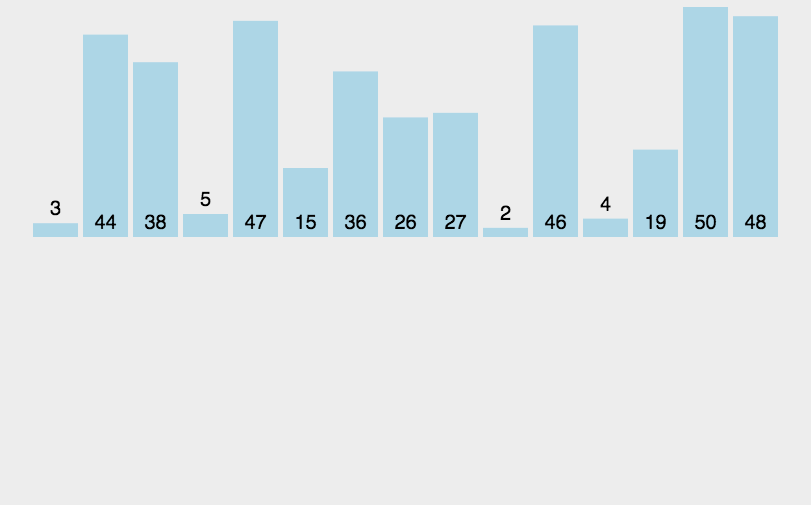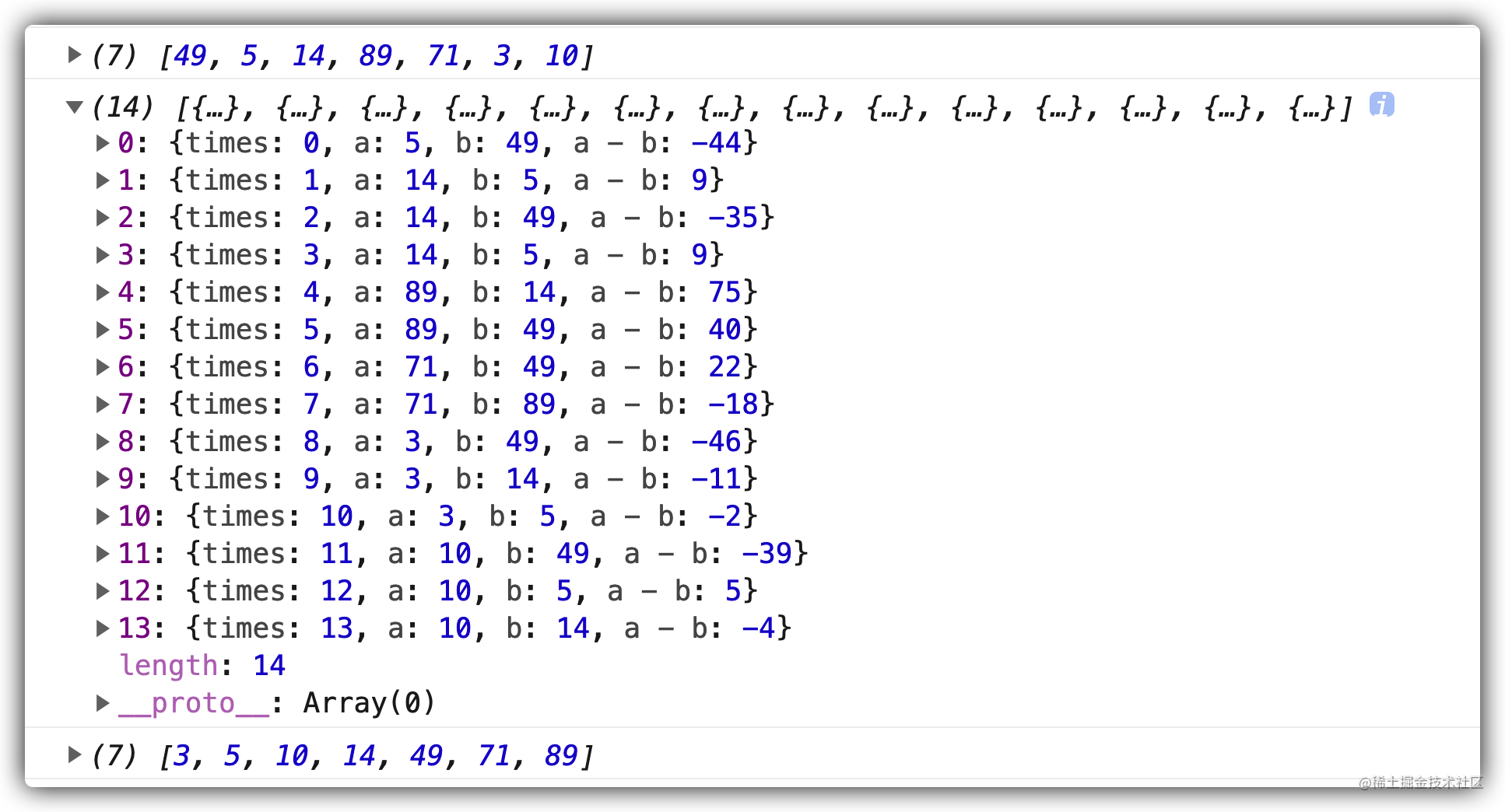# 数组的 sort() 方法详解``````const arr = [49, 5, 14, 89, 71, 3, 10];

// 一般写法
arr.sort(function (a, b) {
return a - b;   // 按照升序排列
});

// 箭头函数
arr.sort((a, b) => a - b);

// 结果  [3, 5, 10, 14, 49, 71, 89]
``````

## 插入排序

• 从第一个元素开始，该元素可以认为已经被排序；
• 取出下一个元素，在已经排序的元素序列中从后向前扫描；
• 如果该元素（已排序）大于新元素，将该元素移到下一位置；
• 重复步骤3，直到找到已排序的元素小于或者等于新元素的位置；
• 将新元素插入到该位置后；
• 重复步骤2~5。## 关于比较函数 `compareFunction`

• 如果 `compareFunction(a, b)` 小于 0 ，那么 a 会被排列到 b 之前。

• 如果 `compareFunction(a, b)` 等于 0 ， a 和 b 的相对位置不变。

• 如果 `compareFunction(a, b)` 大于 0 ， b 会被排列到 a 之前。

• `compareFunction(a, b)` 必须总是对相同的输入返回相同的比较结果，否则排序的结果将是不确定的。## compareFunction 初步探究

``````const arr = [49, 5, 14, 89, 71, 3, 10];
console.log(arr);
let times = 0;
let res = [];
arr.sort((a, b) => {
res.push({times, a, b, "a - b": a - b});
times++;
return a - b;
});
console.log(res);
console.log(arr);
``````• a 的值在按照原数组中的顺序依次变化，这个排序采用的应该是插入排序法
• a 代表的应该是游标
• 从 b 值的变化上又可以看出，a 首次找位置时采用了二分法，a < b 则向前比较， a>b 则向后比较。

### compareFunction 深入探究

``````function InnerArraySort(array, length, comparefn) {
// In-place QuickSort algorithm.
// For short (length <= 22) arrays, insertion sort is used for efficiency.

if (!IS_CALLABLE(comparefn)) {
comparefn = function (x, y) {
if (x === y) return 0;
if (% _IsSmi(x) && % _IsSmi(y)) {
return % SmiLexicographicCompare(x, y);
}
// 将数组元素转换为字符串
x = TO_STRING(x);
y = TO_STRING(y);
if (x == y) return 0;
else return x < y ? -1 : 1;
};
}
var InsertionSort = function InsertionSort(a, from, to) {
for (var i = from + 1; i < to; i++) {
var element = a[i];
for (var j = i - 1; j >= from; j--) {
var tmp = a[j];
// 调用比较函数 a: tmp, b: element
var order = comparefn(tmp, element);
if (order > 0) {
a[j + 1] = tmp;
} else {
break;
}
}
a[j + 1] = element;
}
};

/*** some code here **/

var QuickSort = function QuickSort(a, from, to) {
/*** some code here **/
};
}

function ArraySort(comparefn) {
CHECK_OBJECT_COERCIBLE(this, "Array.prototype.sort");

var array = TO_OBJECT(this);
var length = TO_LENGTH(array.length);
return InnerArraySort(array, length, comparefn);
}

// 源码我就不放了，大家有兴趣研究的话，可以点击上面的链接自行查看
``````

1. v8 中实现 sort() 方法时，采用了 ”插入排序“ 和 ”快速排序“ 两种排序方式。
2. 对于短数组（长度 <= 22）来说，插入排序效率更高。
3. 如果没有传入 `comparefn` ，则生成一个 `comparefn` 比较函数。
4. 在自动生成的比较函数中，会将传入的数组元素通过 `TO_STRING` 方法转换为字符串，再行比较。
5. 对比比较函数中的 b 为游标值，这一点和最新版的 chrome 浏览器表现不同。

``````var order = comparefn(tmp, element);
``````

``````if (order > 0) {
a[j + 1] = tmp;
} else {
break;
}
``````
• 如果返回值`（a-b）`大于0，即 `a > b`, 则将当前拿来比较的值 a 复制给它的下一位，并继续使用游标值 b 向前进行比较。
• 如果返回值小于等于 0 ，则结束比较，并将游标值 b 填在最后一次比较值 a 的后面。

## 总结

``````arr.sort((a,b) => {
const res = a - b;
return res > 0 ? 1 : (res < 0 ? -1 : 0 );
});
``````

~本文完